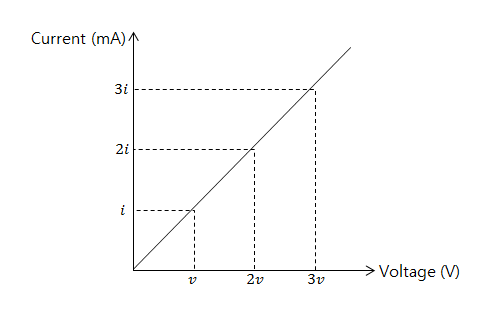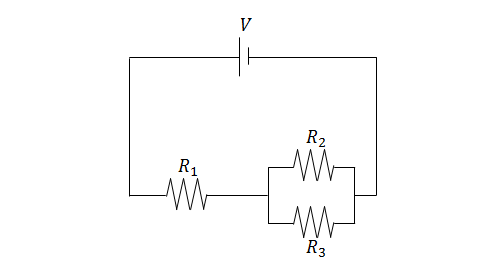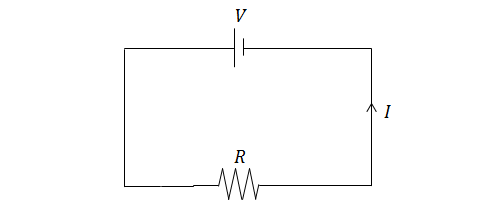Electricity and Magnetism

# Ohm's lawThe above graph shows the current through a nichrome wire according to the voltage applied. If $i=\si{200\ \milli\ampere}$ and $v=\si{125\ \volt}$,) what is the resistance (in $\Omega$) of the nichrome wire?

It is known that the amount of current needed to flow through a human being in order to cause them to have ventricular fibrillation (heart attack) is about 100 mA.

What is the minimum voltage (in volts) that will cause a person who has a body resistance of $1723\ \Omega$ to have ventricular fibrillation?

If a current $I=0.9\text{ A}$ flows through a resistor $R=7\ \Omega$ of length $\Delta d=5\$ m, what is the electric field strength inside the resistor?In the circuit above, $V=5\text{ V},$ $R_1=1\ \Omega,$ $R_2=6\ \Omega,$ and $R_3=12\ \Omega.$ Find the current through $R_1.$If $I=1\text{ A}$ and $R=8\ \Omega,$ what is the voltage of the battery?

×